## Example Questions

### Example Question #1 : How To Find An Angle In A Parallelogram

In a given parallelogram, the measure of one of the interior angles is 25 degrees less than another. What is the approximate measure rounded to the nearest degree of the larger angle?

77 degrees

101 degrees

78 degrees

103 degrees

102 degrees

103 degrees

Explanation:

There are two components to solving this geometry puzzle. First, one must be aware that the sum of the measures of the interior angles of a parallelogram is 360 degrees (sum of the interior angles of a figure = 180(n-2), where n is the number of sides of the figure). Second, one must know that the other two interior angles are doubles of those given here. Thus if we assign one interior angle as x and the other as x-25, we find that x + x + (x-25) + (x-25) = 360. Combining like terms leads to the equation 4x-50=360. Solving for x we find that x = 410/4, 102.5, or approximately 103 degrees. Since x is the measure of the larger angle, this is our answer.

### Example Question #2 : How To Find An Angle In A Parallelogram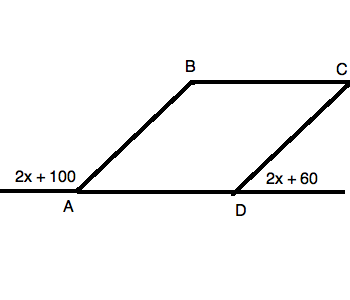Figureis a parallelogram.

Quantity A: The largest angle of.

Quantity B: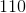Which of the following is true?

Quantity B is larger.

Quantity A is larger.

The relationship cannot be determined.

The two quantities are equal.

The two quantities are equal.

Explanation:

By using the properties of parallelograms along with those of supplementary angles, we can rewrite our figure as follows: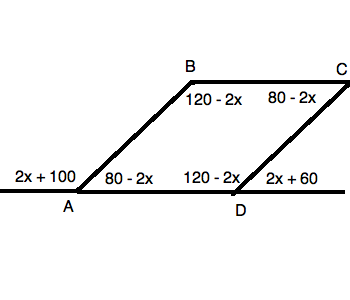Recall, for example, that angle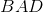is equal to: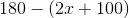, hence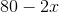Now, you know that these angles can all be added up to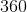. You should also know that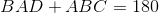Therefore, you can write: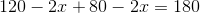Simplifying, you get: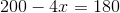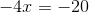Now, this means that: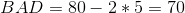and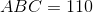. Thus, the two values are equal.

### Example Question #3 : How To Find An Angle In A Parallelogram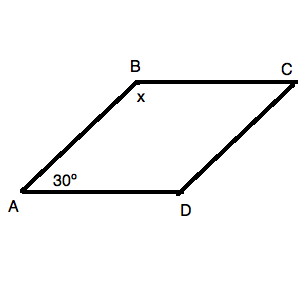Figureis a parallelogram.

What isin the figure above?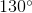Cannot be computed from the data given.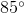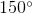Explanation:

Because of the character of parallelograms, we know that our figure can be redrawn as follows: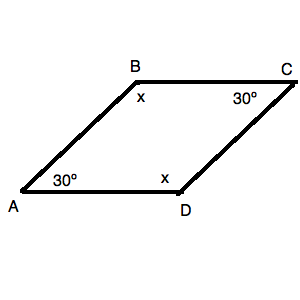Because it is a four-sided figure, we know that the sum of the angles must be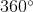. Thus, we know: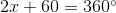Solving for, we get: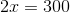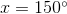Tired of practice problems?

Try live online GRE prep today.